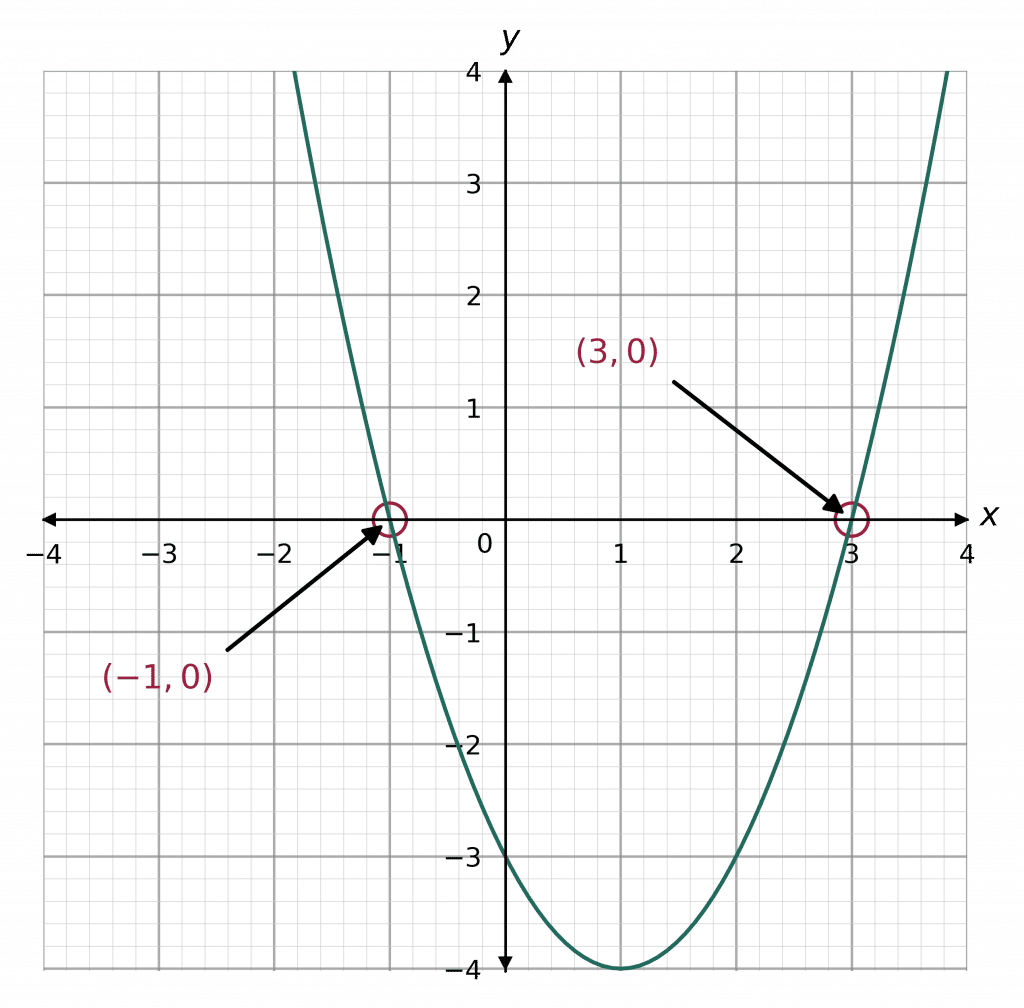GCSELevel 4-5Level 6-7AQAEdexcelEdexcel iGCSEOCRWJEC

## Solving Quadratic Equations by Factorising

Quadratics are algebraic expressions that include the term, $x^2$, in the general form,

$ax^2 + bx + c$

If you are on the foundation course, any quadratic equation you’re expected to solve will always have $a=1$, with all terms on one side and a zero on the other. If you are on the higher course, you may have to do some rearranging in order to get all the terms on one side. Make sure you are happy with the following topics before continuing.

Level 4-5GCSEAQAEdexcelOCRWJECEdexcel iGCSE

## Solving through Factorising ($a=1$)

We can solve quadratics through factorising by following these $4$ easy steps.

Example: Solve the quadratic equation, $\textcolor{blue}{ x^2-3x+2=0}$ by factorisation.

• Step 1: Factorise the quadratic   $\textcolor{blue}{(x -2)(x-1)=0}$
• Step 2: Form two linear equations

Since the right-hand side of the equation is zero, the result of multiplying the two brackets together on the left-hand side must be zero. Therefore, at least one of the brackets must be equal to zero. So, in summary,

If $(x-2)(x-1)=0$, then either $(x-2)=0$ or $(x-1)=0.$

Equation 1:

\begin{aligned}(+2) \,\,\,\,\,\,\,\,\, x - 2 &= 0 \\ x &= 2\end{aligned}

Equation 2:

\begin{aligned}(+1) \,\,\,\,\,\,\,\,\, x - 1 &= 0 \\ x &= 1\end{aligned}

The final roots are:

$x = 2 \,\,\,\,\text{and}\,\,\,\, x=1$

Note: The solutions to a quadratic equation are also called roots, because they correspond to where a quadratic graph crosses the $x$-axis.

Level 4-5GCSEAQAEdexcelOCRWJECEdexcel iGCSE
Level 6-7GCSEAQAEdexcelOCRWJECEdexcel iGCSE

## Solving through Factorising ($a>1$)

Solve the following quadratic equation through factorising $2x^2-3x-9=0$

Step 1: Rearrange the given quadratic so that is it equal to zero ($=0$)

This quadratic is already equal to $0$ so there is nothing more to do.

$(2x+3)(x-3)=0$

Step 3: Form two linear equations

$2x+3=0 \,\,\text{and}\,\, x-3=0$

Step 4: Solve the equations to find the roots of the equation

Equation 1:

\begin{aligned}(-3)\,\,\,\,\,\,\,2x+3&=0 \\ (\div2)\,\,\,\,\,\,\,\,\,\,\,\,\,\,\,\,\,2x&=-3 \\ \,\,\,\,\,\,\,\,\, x& = -\dfrac{3}{2}\end{aligned}

Equation 2:

\begin{aligned}(+3)\,\,\,\,\,\,\,\,\,x-3&=0 \\ x &=3 \end{aligned}

So, the $2$ solutions to the equation are $x=3$ and $x=-\frac{3}{2}$.

Level 6-7GCSEAQAEdexcelOCRWJECEdexcel iGCSE
Level 4-5GCSEAQAEdexcelOCRWJECEdexcel iGCSE

## Quadratic Equations and Sketching Graphs

It is possible to use factorisation to allow you to sketch a quadratic graph.

Example: Use factorisation to find the roots of $x^2 -2x -3=0$ and hence sketch the quadratic.

Like always, the equation needs to be $=0$Step 1: First we need to factorise the left hand side of the equation

$(x-3)(x+1) = 0$

Step 2: Solve the quadratic as show in the above examples

\begin{aligned}(+3)\,\,\,\,\,\,\,\,\,x-3&=0 \\ x&=3\end{aligned}

\begin{aligned}(-1) \,\,\,\,\,\,\,\,\,x+1&=0 \\ \,\,\,\,\,\,\,\,\, x&=-1\end{aligned}

Step 3: Find the coordinates of the roots. We know this equation has the solutions $x= 3$ and $x=-1$

When we set the equation to $0$, $y=0$. This means we can form the two coordinates

$(3,0)$ and $(-1,0)$

Step 4: Identify the $y$-intercept. This is the point at which the curve crosses the $y$-axis and is given by the value of $c$ in the general quadratic $ax^2 + bx +c=0$, which in this case is $-3$.

Once you have found these values, you can sketch the graph, see below.

Note: This graph was done by a computer, but a “sketch” doesn’t have to be perfect, it just has to be the right shape and cross the axes at the right points.

Level 4-5GCSEAQAEdexcelOCRWJECEdexcel iGCSE

## Solving Quadratics Through Factorising Example Questions

The quadratic on the left hand side of the equation factorises so that,

$p^2-3p-10=(p+2)(p-5)$

Therefore, we can rewrite the equation as

$(p+2)(p-5)=0$

For the left-hand side to be zero we require one of the brackets to be zero, hence, the two solutions to this quadratic equation are,

$p=-2$ and $p=5$Gold Standard Education

The quadratic on the left hand side of the equation factorises so that,

$x^2-8x+15=(x-5)(x-3)$

Therefore, we can rewrite the equation as

$(x-5)(x-3)=0$

For the left-hand side to be zero we require one of the brackets to be zero, hence, the two solutions to this quadratic equation are,

$x=5$ and $x=3$Gold Standard Education

To find the roots of a quadratic, we must set it equal to zero and find the solutions of that equation,

$x^2-6x+8=0$

Now, we must factorise. Observing that $(-2)\times(-4)=8$ and $-2+(-4)=-6$, we get that this quadratic factorises to

$(x-2)(x-4)=0$

So, for the left-hand side to be zero we require one of the brackets to be zero.

Therefore, the two roots of this quadratic equation are

$x=2$  and  $x=4$Gold Standard Education

First rearrange the equation so that it equals $0$.

$2x^2+13x+15=0$

The quadratic on the left hand side of the equation factorises so that,

$2x^2+13x+15=(2x+3)(x+5)=0$

For the left-hand side to be zero we require one of the brackets to be zero, hence, the two solutions to this quadratic equation are,

$x=-\dfrac{3}{2}$ and $x=-5$Gold Standard Education

The quadratic on the left hand side of the equation factorises so that,

$(3k-1)(k+6)=0$

So, for the left-hand side to be zero we require one of the brackets to be zero. If the first bracket is zero, then we get

$3k-1=0$

If we add 1 to both sides and then divide by 3, we get the solution

$k=\dfrac{1}{3}$

If the second bracket is zero, then we get

$k=-6$

Therefore, the two solutions to this quadratic equation are,

$k=\frac{1}{3}$ and $k=-6$Gold Standard Education

## Solving Quadratics Through Factorising Worksheet and Example Questions

### (NEW) Solving Quadratics By Factorisation Exam Style Questions - MME

Level 4-5GCSENewOfficial MME

## Solving Quadratics Through Factorising Drill Questions

Level 4-5GCSE

### Solving Quadratics By Factorising (2) - Drill Questions

Level 4-5GCSEProduct

£19.99

/month

Learn an entire GCSE course for maths, English and science on the most comprehensive online learning platform. With revision explainer videos & notes, practice questions, topic tests and full mock exams for each topic on every course, it’s easy to Learn and Revise with the MME Learning Portal.